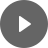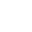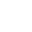8.2 Exponential - StudyNinja
Select Page

HSC Standard Maths Resources

Browse: 1. Home  »  8. Non-linear Relationship »  8.2 Exponential

8.2 Exponential

Exponential curve is formed when the independent variable in an equation is in exponent form.Example 1

Draw the graph of exponential equation y = 3 × 2x + 5. What is the y-intercept and asymptote?

Example 2

What is the value of b and c in the equation related to following graph? Is the value of a greater or less than 1?Get access to 20 Mock Exams with over 700 exam-style questions for HSC Standard Maths.

You might find the following videos helpful related to this section:8A Exponential Functions (1 of 2)

by Mr Bodgers (click to view channel)

An introduction to exponential functions as well as an example where you graph an exponential function using a table of values.8A Exponential Functions (2 of 2)

by Mr Bodgers (click to view channel)

Explains how to perform transformations on exponential graphs.8B Exponential Models (1 of 2)

by Mr Bodgers (click to view channel)

Completes an exponential growth problem based on the exponential growth of the COVID-19 virus in Australia.8B Exponential Models (2 of 2)

by Mr Bodgers (click to view channel)

Completes an exponential decay problem based on the exponential decay of COVID-19 in Australia.The following are the types of questions you can expect in exam:

Study notes of this section and other resources can be accessed here:

Browse: 1. Home  »  8. Non-linear Relationship »  8.2 Exponential